Crystal Symmetries edit page

Crystal symmetries are a sets of rotations and mirroring operations that leave the lattice of a crystal invariant. They form so called groups since the concatenation of two symmetry operations is again a symmetry operation.

Depending which symmetry operations are coonsidered the symmetry groups are categorized either into 230 space groups, 32 point groups or 11 Laue groups.

## Purely enatiomorphic (rotational) symmetry groups

There exist exactly 11 symmetry groups consisting of proper rotations only, namely, 1, 2, 222, 3, 23, 4, 422, 6, 622, 32, 432. These are the so called enatiomorphic groups. All the groups can be defined in MTEX either by its international notation

or by the Schoenflies notation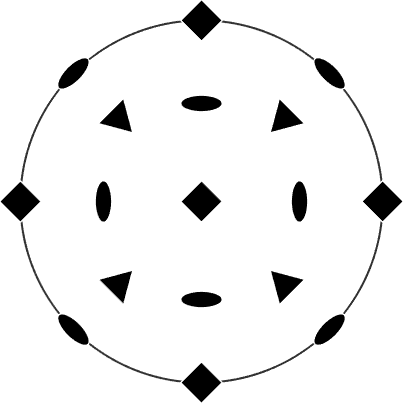## Laue groups

For any symmetry group we obtain the corresponding Laue group by adding the inversion as an additional symmetry element.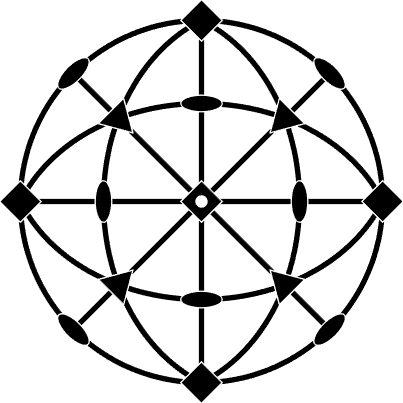More directly, the Laue group corresponding to an arbitrary point group can be defined by the command

Since all Laue groups can be derived from the 11 enantiomorphic groups there are also 11 Laue groups, namely -1, 2/m, mmm, -3, -3m, -4/m, 4/mmm, 6/m, 6/mmm, m-3, m-3m.

The Laue groups have always exactly twice as many symmetry elements as the corresponding enantiomorphic group. As the following example illustrates each symmetry element from the enantiomorphic group occurs two times - with and without inversion - in the corresponding Laue group.

## Point groups

While the enantiomorphic groups contain exclusivly proper rotations and Laue groups contain a proper and an improper version of each rotation, there are also symmetry groups with improper rotations that do not contain the inversion, e.g. the point group mm2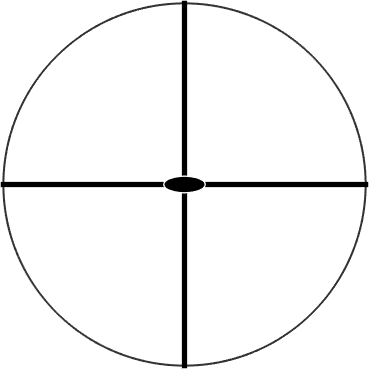We observe that mm2 has exactly the same rotations as 222 with the only difference that half of them are improper. In fact, we can derive all remaining 10 point groups by replacing half of the rotations of a enantiomorphic group by its improper version. This way the following point groups can be constructed: m, mm2, 3m, -4, 4m2, -42m, -6, 6mm, -6m2, -43m. In total this gives us 11 enantiomorphic + 11 Laue + 10 mixed = 32 point groups.

In MTEX we may use the following commands to find the corresponding enantiomorphic group and the corresponding Laue group to any mixed group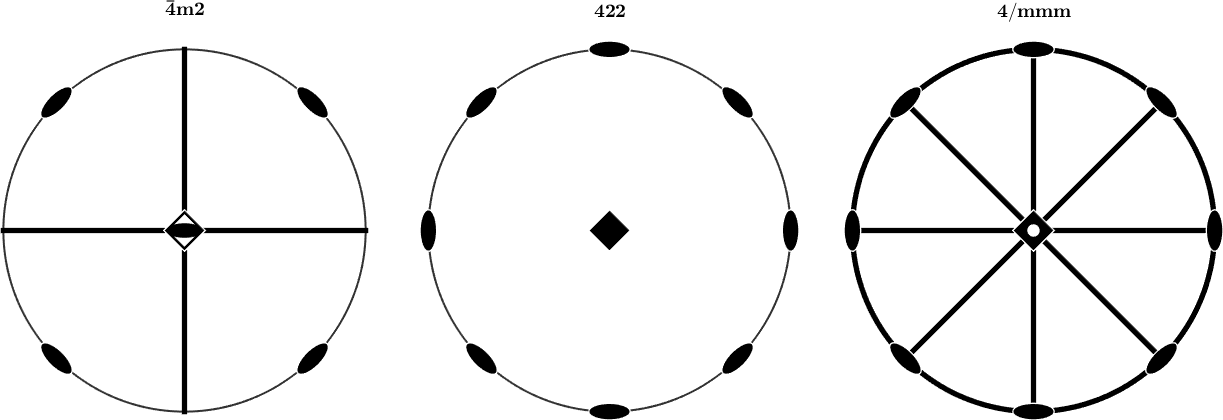## The Subgroup of proper rotations

The enantiomorphic group of a given point group is in general not an subgroup, i.e., it does contain symmetry elements that do not belong to the original point group. If one is interested in the subgroup of proper rotations of a given point group the following command comes into help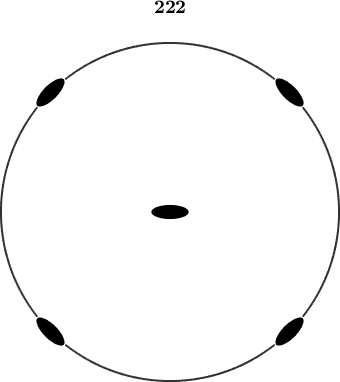## Alignment of the symmetry operations

Although in general only 32 point groups are distingished, some of them allow for different alignments of the symmetry operations with respect to the crystal axes. The following plots show three different alignments of the point group 2mm. Note that the a-axis points in all three case towards south.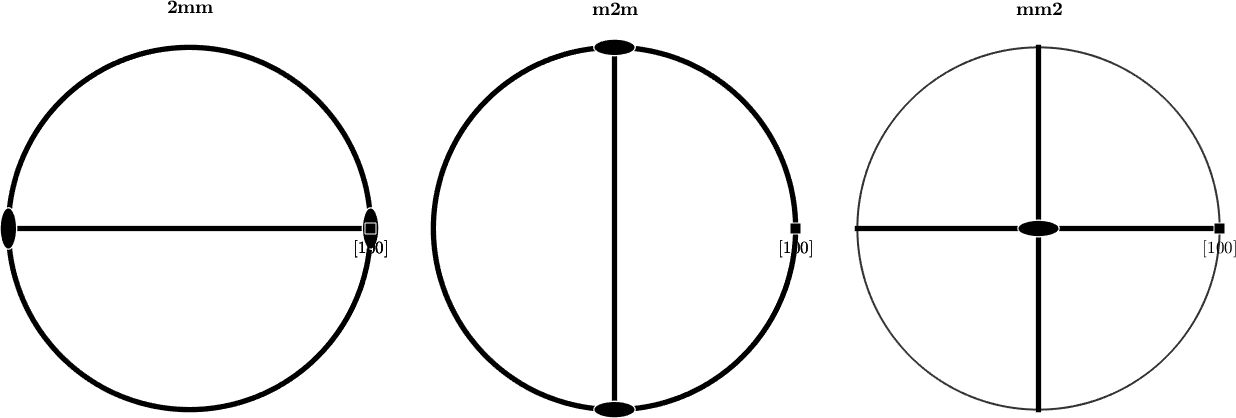Similarly as with mm2, there are different alignements for the point groups 112, 121, 211, 11m, 1m1, m11, 321, 312, 3m1, 31m, etc.

## Space groups

If additionally to the proper and improper rotations also translations are considered as symmetry operations the number of different symmetry groups increases to 320. Those are exhaustivly described in the international table of crystallography.

MTEX currently does not support space groups. If the name of a space group os passed to the command crystalSymmetry, MTEX automatically determines the corresponding point group and assigns it to the variable.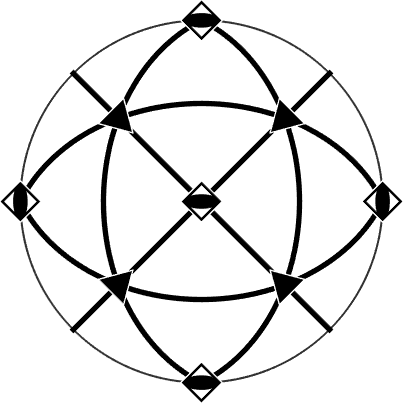## Computations with symmetries

Using the commands union and disjoint new symmetries can be computed from two given ones

## Import from CIF and PHL files

MTEX allows to define a crystal symmetry by importing a crystallographic information file (*.cif)

or a Bruker phl file. As a phl file contains usually many phases the output is a list of crystal symmetries